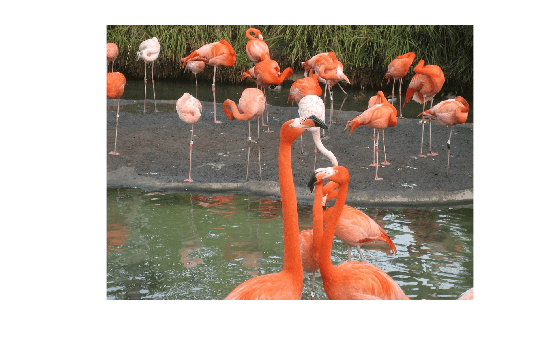randomWindow2d

Randomly select rectangular region in image

Description

example

win = randomWindow2d(inputSize,targetSize) selects a rectangular region of size targetSize from a random position in an image of size inputSize.

example

win = randomWindow2d(inputSize,'Scale',scale,'DimensionRatio',dimensionRatio) selects a rectangular region, specifying the size of the region relative to the input image, scale, and the aspect ratio of the region, dimensionRatio.

Examples

collapse all

imshow(I)Specify the size of the input image and the target size of the rectangular region.

inputSize = size(I);
targetSize = [40 60];

Select a region of the target size from a random location in the image.

rect = randomWindow2d(inputSize,targetSize);

Convert the region from a Rectangle object to a 4-element vector of the form [xmin ymin width height].

rectXYWH = [rect.XLimits(1) rect.YLimits(1) ...
diff(rect.XLimits)+1 diff(rect.YLimits)+1];

Display the boundary of the rectangular region overlaid on the original image.

annotatedI = insertShape(I,"Rectangle",rectXYWH,"LineWidth",3);
imshow(annotatedI)imshow(I)Specify the size of the input image.

inputSize = size(I);

Specify a fractional area of the region between 2% and 13% of the area of the input image. Specify a range of aspect ratios between 1:5 and 4:3.

scale = [0.02 0.13];
dimensionRatio = [1 5;4 3];

Specify a region with a randomly selected fractional area and aspect ratio from a random location in the image.

rect = randomWindow2d(inputSize,"Scale",scale,"DimensionRatio",dimensionRatio);

Crop the original image to the randomly selected region and display the result.

Icrop = imcrop(I,rect);
imshow(Icrop)Input Arguments

collapse all

Input image size, specified as one of the following.

Type of Input ImageFormat of inputSize
2-D grayscale or binary image2-element vector of positive integers of the form [height width]
2-D RGB or multispectral image 3-element vector of positive integers of the form [height width channels]

Target image size, specified as one of the following.

Type of Target ImageFormat of targetSize
2-D grayscale or binary image2-element vector of positive integers of the form [height width]
2-D RGB or multispectral image 3-element vector of positive integers of the form [height width channels]

Region area as a fraction of the input image area, specified as one of these values.

• 2-element nondecreasing numeric vector with values in the range [0, 1]. The elements define a minimum and maximum fractional area of the region, respectively. randomWindow2d selects a random value within the range to use as the fractional region area. To use a fixed region area, specify the same value for both elements.

• Function handle. The function must take no input arguments and return one number in the range [0, 1] specifying a valid fractional region area. For more information about function handles, see Create Function Handle.

Range of aspect ratios of the rectangular region, specified as one of these values.

• 2-by-2 matrix of positive numbers. The first row defines the defines the minimum aspect ratio and the second row defines the maximum aspect ratio. randomWindow2d selects a random value within the range to use as the aspect ratio. To use a fixed aspect ratio, specify identical values for the first and second rows.

• Function handle. The function must take no input arguments and return one positive number specifying a valid dimension ratio. For example, a value of 1.2 specifies a 5:4 aspect ratio. For more information about function handles, see Create Function Handle.

Example: [1 8;1 4] selects an aspect ratio in the range 1:8 to 1:4

Output Arguments

collapse all

Rectangular window, returned as a Rectangle object.